# HSSlive: Plus One & Plus Two Notes & Solutions for Kerala State Board

## BSEB Class 9 Science Chapter 4 Structure of the Atom Textbook Solutions PDF: Download Bihar Board STD 9th Science Chapter 4 Structure of the Atom Book AnswersBSEB Class 9 Science Chapter 4 Structure of the Atom Textbook Solutions PDF: Download Bihar Board STD 9th Science Chapter 4 Structure of the Atom Book Answers

BSEB Class 9th Science Chapter 4 Structure of the Atom Textbooks Solutions and answers for students are now available in pdf format. Bihar Board Class 9th Science Chapter 4 Structure of the Atom Book answers and solutions are one of the most important study materials for any student. The Bihar Board Class 9th Science Chapter 4 Structure of the Atom books are published by the Bihar Board Publishers. These Bihar Board Class 9th Science Chapter 4 Structure of the Atom textbooks are prepared by a group of expert faculty members. Students can download these BSEB STD 9th Science Chapter 4 Structure of the Atom book solutions pdf online from this page.

## Bihar Board Class 9th Science Chapter 4 Structure of the Atom Books Solutions

 Board BSEB Materials Textbook Solutions/Guide Format DOC/PDF Class 9th Subject Science Chapter 4 Structure of the Atom Chapters All Provider Hsslive

## How to download Bihar Board Class 9th Science Chapter 4 Structure of the Atom Textbook Solutions Answers PDF Online?

2. Click on the Bihar Board Class 9th Science Chapter 4 Structure of the Atom Answers.
3. Look for your Bihar Board STD 9th Science Chapter 4 Structure of the Atom Textbooks PDF.
4. Now download or read the Bihar Board Class 9th Science Chapter 4 Structure of the Atom Textbook Solutions for PDF Free.

## BSEB Class 9th Science Chapter 4 Structure of the Atom Textbooks Solutions with Answer PDF Download

Find below the list of all BSEB Class 9th Science Chapter 4 Structure of the Atom Textbook Solutions for PDF’s for you to download and prepare for the upcoming exams:

### Bihar Board Class 9 Science Chapter 4 Structure of the Atom InText Questions and Answers

Page 47

Question 1.
What are canal rays ?
Canal rays are positively charged radiations discovered by E. Goldstein in a discharge tube. These rays led to the discovery of proton. These rays are called canal rays because these rays passed through the holes or canals in the cathode.

Question 2.
If an atom contains one electron and one , proton, will it carry any charge or not ?
No, it will not carry any charge, (The charge will cancel each other).

Page 49

Question 1.
On the basis of Thomson’s model of an atom, explain how the atom is neutral as a whole.
In 1899, Thomson proposed that:
(i) An atom consists of a positively charged sphere and the electrons are embedded in it.
(ii) The negative and positive charges balance each other and so the atom as a whole is neutral.

Question 2.
On the basis of Rutherford’s model of an atom, which sub-atomic particle is present in the nucleus of an atom.
On the basis of Rutherford’s model of an atom proton is present in the nucleus of an atom. ‘

Question 3.
Draw a sketch of Bohr’s model of an atom with three shells.Question 4.
What do you think would he the observation if the a-particle scattering experiment is carried out using a foil of a metal other than gold ?
Gold is the most malleable metal and hence it increases the probability of the a-particle hitting the atom in the first plane. Also, gold is the least reactive metal. If any other metal is used other than gold, the metal may react with the a-partieles or the a-particles may not be able to hit the atom if the metal is thick and less malleable.

Page 49

Question 1.
Name the three sub-atomic particles of an atom.
The three sub-atomic particles of an atom are, electrons, protons and neutrons.

Question 2.
Helium atom has an atomic mass of 4 u and two protons in its nucleus. How many neutrons does ithave?
Atomic mass of Helium atom = 4u
Number of protons = 2
Number ofneutrons = 2 (4 – 2 = 2)

Page 50

Question 1.
Write the distribution of electrons in carbon and sodium atoms.
(i) Atomic number of carbon = 6
Distribution of electrons =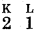(ii) Atomic number of sodium = 11
Distribution of electrons =Question 2.
If K and L shells of an atom are foil, then what would be the total number of electrons in the atom ?
If K and L shells of an atom are full, then the total number of electrons in the atom would be 10. (K-shell can have maximum 2 electrons and L shell can have maximum 8 electrons.)

Page 52

Question 1.
How will you find the valency of-
(i) Chlorine –
(ii) Sulphur
(iii) Magnesium.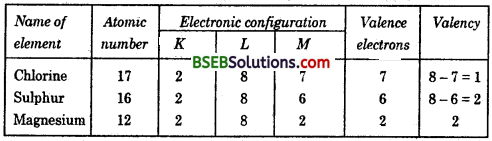Page 52

Question 1.
If number of electrons in an atom is 8 and number of protons is also 8, then
(i) what is the atomic number of the atom ?
(ii) what is the charge on the atom ?
(i) Atomic number = Number of protons = 8.
(ii) Electronic configuration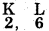.’. Charge on the atom will be 2+.

Question 2.
With the help of table find out the mass numbers of oxygen and sulphur atom.Mass number = No. of protons + No. of neutrons
Mass number of oxygen = 8 + 8 = 16
Mass number of sulphur = 16 + 16 = 32.

Page 53

Question 1.
For the symbol H, D and T tabulate three sub-atomic particles found in each of them.
There are three isotopes of hydrogen :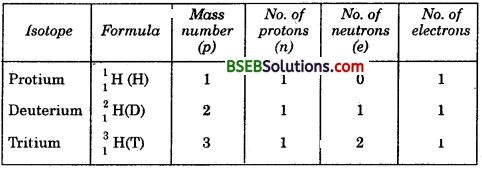Question 2.
Write the electrbnic configuration of any one pair of isotopes and isobars.
(i) Isobars : 4020Ca and 4018Ar
Electronic configuration of Ca = 2,8,8,2.
Electronic configuration of Ar = 2,8, 8.
(ii) Isotopes : 126C and 146C .
Electronic configuration of C = 2, 4.

### Bihar Board Class 9 Science Chapter 4 Structure of the Atom Textbook Questions and Answers

Question 1.
Compare the properties of electrons, protons and neutrons.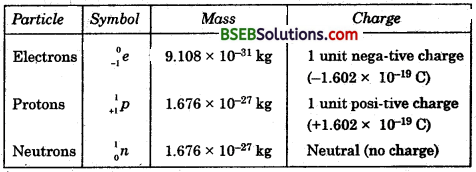Question 2.
What are the limitations of J. J. Thomson’s model of the atom ?
Although Thomson’s model explained well that atoms are electrically neutral, but the results of experiments done by other scientists could not be explained by this model.

Question 3.
What are the limitations of Rutherford’s model of the atom ?
The major drawback of Rutherford’s model of an atom is that it does not explain the stability of an atom. When charged bodies move in circular orbits, they emit radiation. This emission of radiation would cause a reduction in the energy of the electrons. The electrons should come closer and closer to the nucleus, as they lose energy and finally fall into the nucleus. Rutherford could not explain why this did not seem to be happening.

Question 4.
Describe Bohr’s model of the atom.
A Danish physicist, Neils Bohr proposed an atomic model in 1913. This model of atom is called Bohr’s model of atom.
Basic postulates of the Bohr’s atomic model are :
(i) In an atom, the electrons revolve around the nucleus in certain definite circular orbits. These circular orbits are also called energy shells, or energy levels.
(ii) An electron revolving in a particular orbit has a fixed energy.
(iii) The orbits, or the energy shells (Fig. 4.10) are numbered as n = 1, 2, 3, 4… etc., or K, L, M, N… etc., shells starting from the nucleus. These integers 1, 2, 3, 4, … etc., are known as quantum numbers of the orbits.
(iv) An electron can lose or gain only certain definite energies.

Question 5.
Compare all the proposed models of an atom given in this chapter.Question 6.
Summarize the rules for writing of distribution of electrons in various shells for the ‘ first eighteen elements.
Bohr and Bury scheme for the distribution of electrons in an atom is based on the following rules :
(i) The maximum number of electrons which a shell can have is 2n2, where n is the quantum number of that energy shell. So, the maximum number of electrons in various shells are,
1st (K) shell 2 x 12 = 2
2nd (L) shell 2 x 22 = 8
3rd (M) shell 2 x 32 = 18
4th (N) shell 2 x 42 = 32
(ii) The outermost shell (also called valence shell) can have a maximum of 8 electrons. Next to the outermost shell (called penultimate shell) can accommodate a maximum of 18 electrons, if permitted by rule (i).
(iii) Electrons are not accommodated in a given shell unless the inner shells are filled. That is the shells are filled in a step-wise manner.

Question 7.
Define valency by taking examples of silicon and oxygen.
The combining capacity of an atom of an element is known as its valency. The number of electrons gained, lost or shared so as to make the octet of electrons in the outermost shell of an atom of an element gives us directly the combining capacity of the element.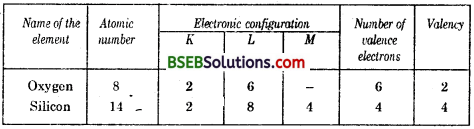Valency of oxygen is 2 because it can complete its octet by gaining 2 electrons. Valency of silicon is 4 because it can loose, gain or share 4 electrons to complete its octet.

Question 8.
(a) Explain with examples :
(i) Atomic number
(ii) Mass number
(iii) Isotopes
(iv) Isobars.
(b) Give any two uses of isotopes.
(a) (i) The number of protons present in the nucleus of an atom of an element is called its atomic number.
For hydrogen, Z = 1 because in hydrogen atom only one proton is present in the nucleus.
For carbon, Z = 6 because in carbon atom six protons are present in the nucleus.

(ii) The sum of the total number of protons and neutrons present in the nucleus of an atom of an element is called mass number. For example, Mass of carbon is 12 because it has 6 protons and 6 neutrons (6 + 6 = 12). Similarly, mass number of aluminium is 27 (13 p + 14 n).

(iii) Atoms of the same element, having the same atomic number, but different mass numbers, are called isotopes of that element.

Isotopes may also be defined as follows :
“The atoms of an element having the same number of protons, djot different number of neutrons are called isotopes of that element.”
Examples :
(i) There are three isotopes of hydrogen :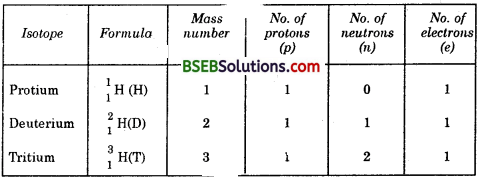(ii) There are two isotopes of chlorine.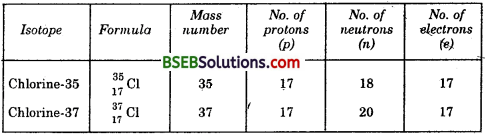(iii) Atoms of those elements which have same mass numbers but different atomic numbers are known as isobars. For example,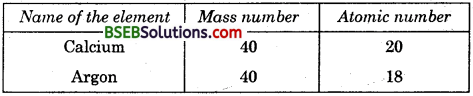(b) Some applications of isotopes are :
(i) An isotope of Uranium is used as a fuel in a nuclear reactor.
(ii) An isotope of Cobalt is used in the treatment of cancer.
(iii) An isotope of Iodine is used in the treatment of goitre.

Question 9.
Na+ has completely filled K and L shells. Explan.
The atomic number of sodium is 11.
Distributio of electronsA positively charged ion is formed by loosing elecrons. The sodium atom looses one electron and forms sodium ion (Na+). The distribution of electrons in sodium ion is 2 8. Thus, Na+ has completely filled K and L shells since the maximum capacity of K shell is 2 and the maximum capacity of L shell is 8.

Question 10.
If bromine atom is available in the form of, say, two isotopes 7535Br (49.7%) and 8135Br (50.3%), calculate the average atomic mass of bromine’ atom.
The atomic mass of bromine atom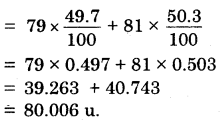Question 11.
The average atomic mass of a sample of an element X is 16.2u. What are the percentage of isotopes 168X and 188X in the sample?
Let, the percentage of 168 X in the sample be x. Then,
the percentage of 188 X in the sample is (100 – x). Then
atomic mass of X = 16 x (𝑥100) + 18 x (100–𝑥100)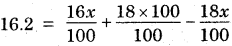1620 = 16x – 18x + 1800
or, 2x = (1800 – 1620) = 180
Thus, x = 180/2 = 90
Percentage of X in the sample = 90
Percentage ofX in the sample = 10:

Question 12.
If Z = 3. what would be the valency of the element ? Also, name the element.
Atomic number, Z = 3

Distribution of electrions =∵ Valency = 1
The element is Lithium.

Question 13.
Composition of the nuclei of two atomic species X and Y are given as under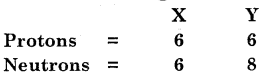Give the mass number of X and Y. What is the relation between the two species ?
Mass number = Number of protons + Number of neutrons
Mass number of X = 6 + 6 = 12
Mass number of Y = 6 + 8 = 14
Atomic number = Number of protons.
Atomic number of X= 6
Atomic number of Y = 6.
Since X and Y have the same atomic number but different mass number they are isotopes.

Question 14.
For the following state-ments write T for true and F for false.
(а) J.J. Thomson proposed that the nucleus of an atom contains only nucleons.
(b) A neutron is formed by an electron and a proton combining together. Therefore it is neutral.
(c) The mass of an electron is about 12000 times that of a proton.
(d) Isotope of iodine is used for making tincture iodine which is used as a medicine.
a, b, c, d = False
e = True
Put tick against the correct choice in Q. 15, 16 and 17.

Question 15.
Rutherford’s alpha-particle scattering experiment was responsible for the discovery of
(a) Atomic Nucleus
(b) Electron
(c) Proton
(d) Neutron.
(a) Atomic nucleus.

Question 16.
Isotopes of an element have
(a) the same physical properties
(b) different chemical properties
(c) different number of neutrons
(d) different atomic numbers.
(c) Different number of neutrons.

Question 17.
Number of valence electrons in Cl ion are :
(a) 16
(b) 8
(c) 17
(d) 18
(6) 8.

Question 18.
Which of the following is a correct electronic configuration of sodium ?
(a) 2, 8
(b) 8, 2,1
(c) 2,1, 8
(d) 2, 8,1.
(d) 2, 8, 1.

Question 19.
Complete the following table :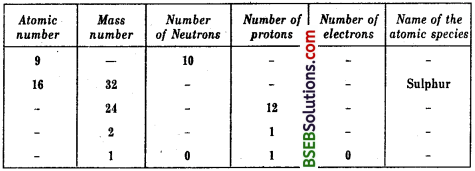### Bihar Board Class 9 Science Chapter 4 Structure of the Atom Textbook Activities

Activity 4.1

(A) Comb dry. Does the comb then attract small pieces of paper ?
(B) Rub a glass rod with a silk cloth and bring the rod near an inflated balloon. Observe what happens.
(C)What can you conclude from these activities ?
(A) The comb will attract small pieces of paper.
(B) The inflated balloon will stick to the glass rod.
(C) We can conclude that on rubbing two articles together, they become electrically charged.

Activity 4.2

Make a static atomic model displaying electronic configuration of the first eighteen elements.
The model can be made by seeing Fig. 4.12. Use plaster cine/plaster of paris/clay and wool/wire to make the model.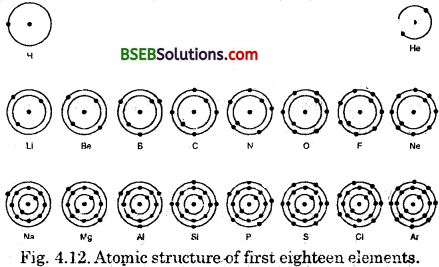### Bihar Board Class 9 Science Chapter 4 Structure of the Atom Additional Important Questions and Answers

Question 1.
Name the fundamental building blocks of matter. –
Atoms and molecules are the fundamental building blocks of matter. –

Question 2.
How is an electron repre-sentcd ?
An electron is represented as e+ or e

Question 3.
Name the scientist who dis-covered proton.
E. Goldstein discovered proton.

Question 4.
How is a proton represented ?
A proton is represented as ‘p’ or p+.

Question 1.
Name the fundamental building blocks of matter.
Atoms and molecules are the fundamental building blocks of matter.

Question 2.
What is the cause of existence of different kinds of matter ?
The existence of different kinds of matter is due to different atoms constituting them.

Question 3.
What was the major challenge before the scientists at the end of the 19th century ?
The major challenge was to reveal the structure of atom as well as explain its important properties.

Question 4.
What is the elucidation of structure of atoms based on ?
The elucidation of structure of atoms is based on a series of experiments performed between 1895 to 1905.

Question 5.
From where do we get the first indication that atoms are not indivisible ?
Anser:
One of the first indication that atoms are not indivisible comes from studying static electricity and the condition under which electricity is conducted by different substances.

Question 1.
Describe the properties of the cathode rays.
(i) Cathode rays travel in straight lines. That is why, these rays cast the shadow of any solid object placed in their path (Fig. 4.4).(ii) Cathode rays consist of material particles. The cathode rays can rotate a paddle wheel when placed in the path of these rays (Fig. 4.5). This shows that the cathode rays consist of stream of material particles.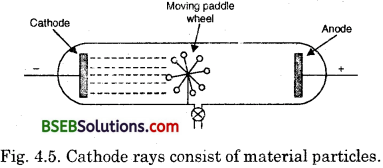(iii) Cathode rays consist of negatively charged particles. Cathode rays when placed in an electrical field get deflected towards the positive plate (Fig. 4.6).(iv) Cathode rays travel with high speeds approaching that of light.
(v) Cathode rays ionise the gas through which they pass.
(vi) Cathode rays are deflected by the magnetic fields.
(vii) Cathode rays can penetrate through thin metallic sheets.
(viii) When cathode rays fall on solids, such as copper, X-rays are produced.
(ix) Cathode rays heat the object on which they fall, due to the transfer of kinetic energy.
(x) The surface on which the cathode rays fall show a coloured shine.
(xi) The nature of cathode rays, (e.gcharge and mass) does not depend upon the nature of the gas inside the tube, and the material used for making the cathode.

## Bihar Board Class 9th Science Chapter 4 Structure of the Atom Textbooks for Exam Preparations

Bihar Board Class 9th Science Chapter 4 Structure of the Atom Textbook Solutions can be of great help in your Bihar Board Class 9th Science Chapter 4 Structure of the Atom exam preparation. The BSEB STD 9th Science Chapter 4 Structure of the Atom Textbooks study material, used with the English medium textbooks, can help you complete the entire Class 9th Science Chapter 4 Structure of the Atom Books State Board syllabus with maximum efficiency.

## FAQs Regarding Bihar Board Class 9th Science Chapter 4 Structure of the Atom Textbook Solutions

#### Can we get a Bihar Board Book PDF for all Classes?

Yes you can get Bihar Board Text Book PDF for all classes using the links provided in the above article.

## Important Terms

Bihar Board Class 9th Science Chapter 4 Structure of the Atom, BSEB Class 9th Science Chapter 4 Structure of the Atom Textbooks, Bihar Board Class 9th Science Chapter 4 Structure of the Atom, Bihar Board Class 9th Science Chapter 4 Structure of the Atom Textbook solutions, BSEB Class 9th Science Chapter 4 Structure of the Atom Textbooks Solutions, Bihar Board STD 9th Science Chapter 4 Structure of the Atom, BSEB STD 9th Science Chapter 4 Structure of the Atom Textbooks, Bihar Board STD 9th Science Chapter 4 Structure of the Atom, Bihar Board STD 9th Science Chapter 4 Structure of the Atom Textbook solutions, BSEB STD 9th Science Chapter 4 Structure of the Atom Textbooks Solutions,
Share: